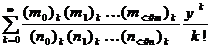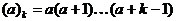>>  <<  Usr  Pri  JfC  LJ  Phr  Dic  Rel  Voc  !:  Help  J for C Programmers

31. Applied Mathematics in J

Numbers in radix form comprise a multiplier, a base, and an exponent, written together with no spaces; the value of the number is multiplier * base ^ exponent .  The base is indicated by a letter, chosen from e (base 10), p (base π, 3.14159...), or x (base e, 2.71828...).  Examples:

1p1

3.14159

1x1

2.71828

1e3

1000

Complex Numbers

All the mathematical functions can be applied to complex numbers.  A complex constant is written with the letter j separating the real and imaginary parts, e. g. 0j1 is the square-root of _1 .  Alternatively, a constant can be written in polar form in which the letters ar (or ad) separate the magnitude of the number from the angle in radians (or degrees) between the real axis and a line in the complex plane from the origin to the point representing the number:

1ar1

0.540302j0.841471

0j1

A number of verbs are available for operations on components of complex numbers.  All have rank 0.

+ y    conjugate of y

+. y    creates a 2-atom list of the real and imaginary components of y

. y    creates a 2-atom list of the length and angle in radians of the polar form of y

| y    magnitude of y

j. y    0j1 * y

r. y    ^ 0j1 * y

x j. y    x + j. y (i. e. x is the real part, y is the imaginary part)

x r. y    x * r. y (i. e. polar form, where x is the magnitude and y the angle in radians)

! y    factorial of y (more generally, the gamma function Γ(1+y))

Matrix Operations

J has primitive verbs for operations on matrices, some using the conjunction .  -/ .* y gives the determinant of y; x +/ .* y is the matrix product of x and y; %. y is the matrix inverse of y (provided y does not have dependent columns); x %. y is the projection of x onto the column space of .

If y is square, %. y is y-1, the inverse matrix of y .  If y is not square, it must have more rows than columns, and %. y is (yTy)-1yT .

x %. y is (%. y) +/ .* x .

In x +/ .* y, a rank-1 x is treated as a matrix with one row, and a rank-1 y is treated as a matrix with one column; but the result rank, which is 2 when rank-2 matrices are multiplied, is 1 when one operand has rank 1 and 0 when both do.

x %. y is a rough-and-ready way to get a least-squares approximation of x as a linear combination of the columns of .  If your y is singular or close to it, avoid %. and use methods based on singular value decomposition.

J supports 3 different ways of specifying a polynomial:

2.      as a list of coefficients of increasing powers, starting with power 0 (i. e. a constant term), where each coefficient multiplied by the corresponding power of the variable produces a term; the polynomial is the sum of the terms.  This form is an unboxed numeric list.

3.      as a multiplier m and a list of roots r, representing the polynomial m(x-r0)(x-r1)...(x-rn).  This form is a 2-item list of boxes m;r (if m is 1 it may be omitted, leaving just <r).

4.      (for multinomials) a multinomial of n variables is represented as a list of terms, each term consisting of a coefficient and a list of n exponents (one exponent per variable).  The multinomial is the sum of the terms.  The form is a boxed rank-2 array in which each item is a coefficient followed by the list of n exponents.  This form is distinguished from the multiplier-root form by the rank of the boxed array.  (It is possible to have multiple multinomials that share a common exponent array, by having more than one coefficient preceding each list of exponents, but we will not pursue that here)

For example, three ways of expressing the polynomial 3x3-12x (which can be written 3x(x+2)(x-2))are 0 _12 0 3, 3;_2 0 2, and <2 2\$_12 1 3 3 .

p. y has rank 1 and converts between coefficient and multiplier-root form of the polynomial .  Note that converting from coefficient form to multiplier-root form solves for the roots of the polynomial.

p. 0 _12 0 3

+-+------+

|3|2 _2 0|

+-+------+

p. 3;2 0 _2

0 _12 0 3

If the multinomial form has only one variable (i. e. each item has length 2), monad p. will convert it to coefficient form:

p. <2 2\$_12 1 3 3

0 _12 0 3

Dyad p. has rank 1 0 and is used to evaluate a polynomial in any of these forms.  If x is a polynomial in coefficient or multiplier-root form, x p. y evaluates it with y giving the value of the variable:

0 _12 0 3 p. 1

_9

(3;_2 0 2) p. _2 _1 0 1 2

0 9 0 _9 0

(the second evaluation applied the polynomial to 5 different values of the variable).

If x is a multinomial, x p. <y evaluates it with the list y giving the values of the variables (y must be boxed because the right rank of dyad p. is 0 and in this case there is more than one variable).  So to evaluate the binomial x3+3x2y+3xy2+y3 with x=2 and y=3 we have

(<4 3\$1 3 0  3 2 1  3 1 2  1 0 3) p. <2 3

125

as expected.

Calculus: d., D., D:, and p..

J provides support for differential and integral calculus.  u d. n produces the verb that gives the nth derivative of :

*: d. 1

+:

*: y is y squared; the derivative is +: y which is y doubled.

^&3 d. 1

3&*@(^&2)

^&3 y is y cubed; the derivative is 3 * y ^ 2 .

^&3 d. 2

3"0 * +:

Second derivative is 3 * 2 * y .

*: d. _1

0 0 0 0.33333&p.

The _1st derivative is the indefinite integral, which is (y ^ 3) % 3 .  The form the interpreter uses is the polynomial form.

f =. *:

f d. _1 (6)

72

g =. *:@+:

g d. _1 (6)

288

u d. n produces ordinary derivatives, and evaluates its u with rank 0.  For partial derivatives, use u D. n where u has rank greater than 0.  Each cell of y produces an array of results, one result for each atom of the cell, giving the partial derivative with respect to that atom.  For example, the length of a vector is given by

veclength =: +/&.:*:"1

which squares the atoms, adds them, and takes the square root:

veclength 3 4 5

7.07107

The derivative of the vector length with respect to the individual components is given by:

veclength D. 1 (3 4 5)

0.424264 0.565685 0.707107

The result of u need not be a scalar.  Here we define the cross product:

xp =: dyad : '((1|.x)*(_1|.y)) - ((_1|.x)*(1|.y))'"1

0 0 2&xp D. 1 (4 2 0)

0 2 0

_2 0 0

0 0 0

Each row is the vector-valued partial derivative of the cross product (0 0 2 xp 4 2 0) with respect to one component of .

The interpreter will bend every effort to find a derivative for your function, but it may fail, or you may not like the derivative it chooses.  m D. n, when m is a gerund u`v, produces a new verb which executes like u but whose nth derivative is :

a =. *:`] D. 1

Here a is defined to be *: except that its derivative is .

a

*:`]D.1

a D. 1 (5)

5

Sure enough, the derivative is .

If you don't want to express the derivative, you can have the interpreter approximate it for you.  x u D: n y approximates the derivative at y by evaluating u at y and y+x .  Both the left and right ranks of u D: n are the monadic rank of u, so you can specify different step-sizes for different atoms of y (if x is a scalar, it is used for the step-size at all atoms of y).

You can do calculus on polynomials by manipulating the polynomial forms without having to create the verbs that operate on those forms.  p.. y (rank 1) takes polynomial y in either coefficient or multiplier-root form and produces the coefficient form of the derivative of x p.. y (rank 0 1) produces the coefficient form of the integral of y with constant term .

Taylor Series: t., t:, and T.

With derivatives available, Taylor series are a natural next step.  u t. y is the yth Taylor coefficient of u expanded about 0, and x u t. y evaluates that term at the point x, in other words x u t. y is x^y * u t. y .  All ranks of u t. are 0.

u T. n is a verb which is the n-term Taylor approximation to u (expanded about 0).

u t: y is (!y) * u t. y .

The generalized hypergeometric function is specified by two lists of numbers, a numerator list and a denominator list.  The generalized hypergeometric function is the sum over all k of the (infinite) generalized hypergeometric series, which is a power series in which the coefficient of the yk term is the product of the rising factorials of length k of the numerator items divided by a similar product for the denominator items, and then divided by !k .

The conjunction H. is used in the form m H. n where m is the numerator list and n is the denominator list.  The resulting verb m H. n has rank 0.  The monad m H. n y takes the limit of the sum of the generalized hypergeometric series; the dyad x m H. n y takes the sum of the first x terms.  Formally, the generalized hypergeometric function iswhereIf m contains 2 items and n contains 1 item, m H. n defines a hypergeometric function.

Generalized hypergeometric functions can be used to calculate a great many functions of interest: Legendre polynomials, Laguerre polynomials, Chebyshev polynomials, and Bessel functions of the first kind are all special cases of hypergeometric functions.  Ewart Shaw, in http://www.ewartshaw.co.uk/data/jhyper.doc, gives a number of examples of uses of H. .  For example, the error function and the cumulative distribution function are given by

erf =: 3 : '(((2p_0.5)*y) % (^*:y)) * 1 H. 1.5 *: y'

n01cdf =: 3 : '-: >: erf y % %:2'   NB. CDF of N(0,1)

where I have rewritten Shaw's formulas to use elementary J.  2p_0.5 is 2/sqrt(π).

\$. y converts the array y into a sparse-matrix representation which can save a lot of space and time if most of the atoms of y have the same value.  \$.^:_1 y converts sparse y back to normal (dense) form.  A large but incomplete subset of operations is supported on sparse arrays; look at the description of \$. if you think you'd like to use them.

Monad ? has rank 0.  If y is 0, ? y is a random floating-point number uniformly distributed in the interval 0 <= ? y < 1.  If y is positive, ? y is a random element of i. y .  An example use is

? 3 3 \$ 1000

755 458 532

218  47 678

679 934 383

Dyad ? has rank 0.  x ? y is a list of x items selected without repetition from i. y, as if the list i. y were shuffled and the first x elements were taken:

5 ? 52

24 8 48 46 22

The ? verbs use the Mersenne Twister generator by default.  Foreigns, described.in the Dictionary page for ?, allow selection of other generators.

The web site at www.jsoftware.com has several addons that you can download.  These are executable libraries, along with J scripts to call functions in them, that offer efficient implementations of often-used functions.  Two of interest in applied mathematics are the LAPACK addon and the FFT addon.  If you want a fast implementation of the singular value decomposition referred to earlier, install the LAPACK addon; then you can use